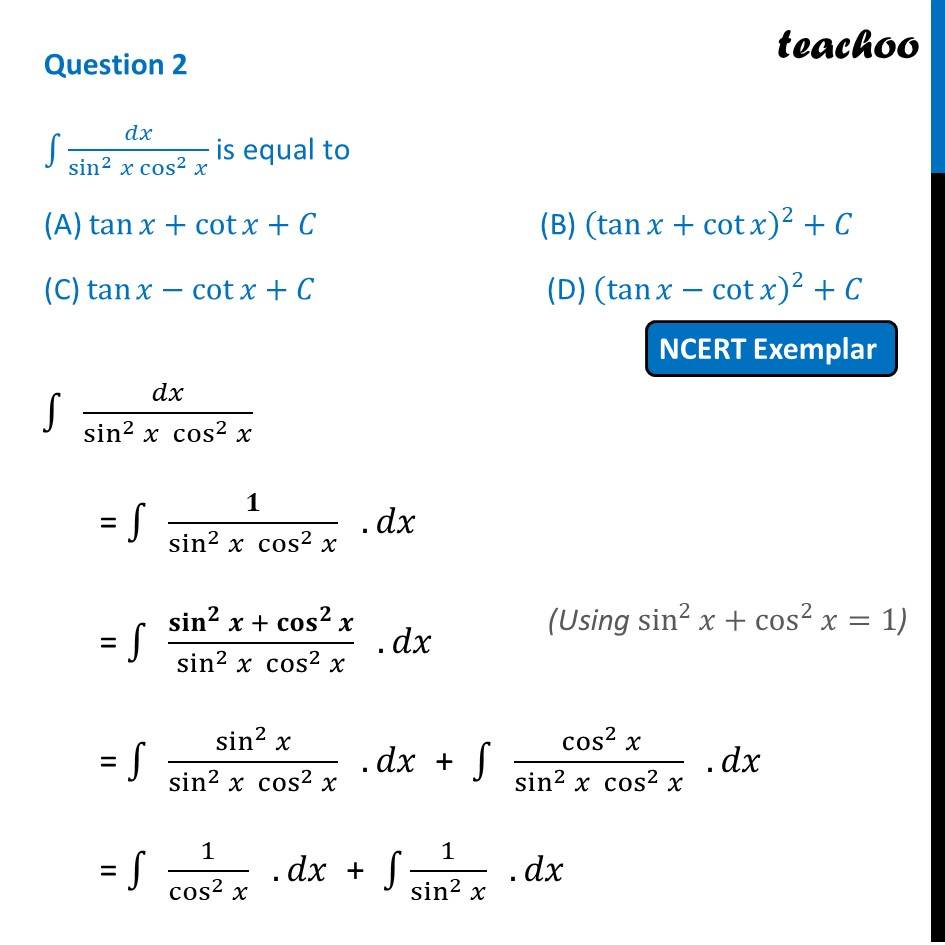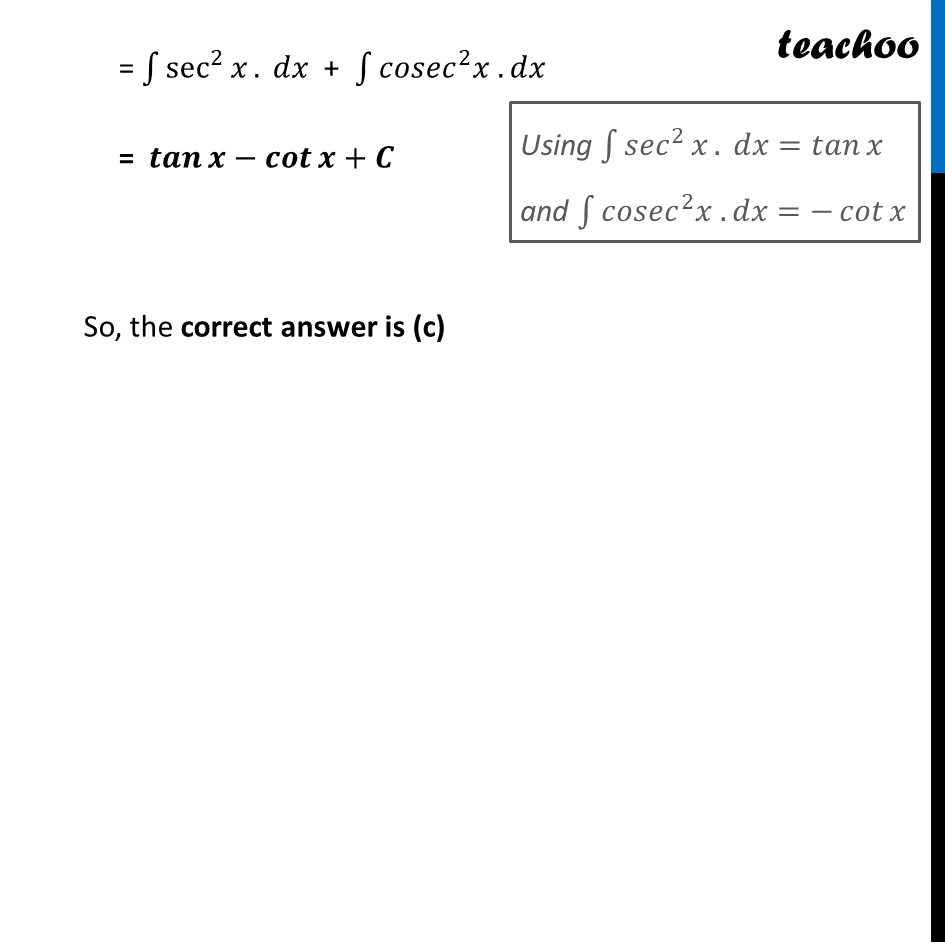NCERT Exemplar MCQ

Chapter 7 Class 12 Integrals
Serial order wise

## (D) (tan⁡x - cot⁡x )^2 + C

This question is similar to Ex 7.2, 39 (MCQ) - Chapter 7 Class 12 - IntegralsLearn in your speed, with individual attention - Teachoo Maths 1-on-1 Class

### Transcript

Question 2 ∫1▒𝑑𝑥/(sin^2⁡𝑥 cos^2⁡𝑥 ) is equal to (A) tan⁡𝑥+cot⁡𝑥+𝐶 (B) (tan⁡𝑥+cot⁡𝑥 )^2+𝐶 (C) tan⁡𝑥−cot⁡𝑥+𝐶 (D) (tan⁡𝑥−cot⁡𝑥 )^2+𝐶 ∫1▒〖" " 𝑑𝑥/(sin^2 𝑥 cos^2⁡𝑥 )〗 = ∫1▒〖" " 𝟏/(sin^2 𝑥 cos^2⁡𝑥 ) . 𝑑𝑥〗 = ∫1▒〖" " (〖𝐬𝐢𝐧〗^𝟐 𝒙 +〖 〖𝐜𝐨𝐬〗^𝟐〗⁡𝒙)/(sin^2 𝑥 cos^2⁡𝑥 ) . 𝑑𝑥〗 = ∫1▒〖" " (sin^2 𝑥)/(sin^2 𝑥 cos^2⁡𝑥 ) . 𝑑𝑥〗 + ∫1▒〖" " 〖 cos^2〗⁡𝑥/(sin^2 𝑥 cos^2⁡𝑥 ) . 𝑑𝑥〗 = ∫1▒〖" " 1/cos^2⁡𝑥 . 𝑑𝑥〗 + ∫1▒〖1/(sin^2 𝑥) . 𝑑𝑥〗 = ∫1▒〖sec^2⁡𝑥. 𝑑𝑥〗 + ∫1▒〖𝑐𝑜𝑠𝑒𝑐^2 𝑥 . 𝑑𝑥〗 = 𝒕𝒂𝒏⁡𝒙−𝒄𝒐𝒕⁡𝒙+𝑪 So, the correct answer is (c)